# Lorentz Transformation Equation & Derivation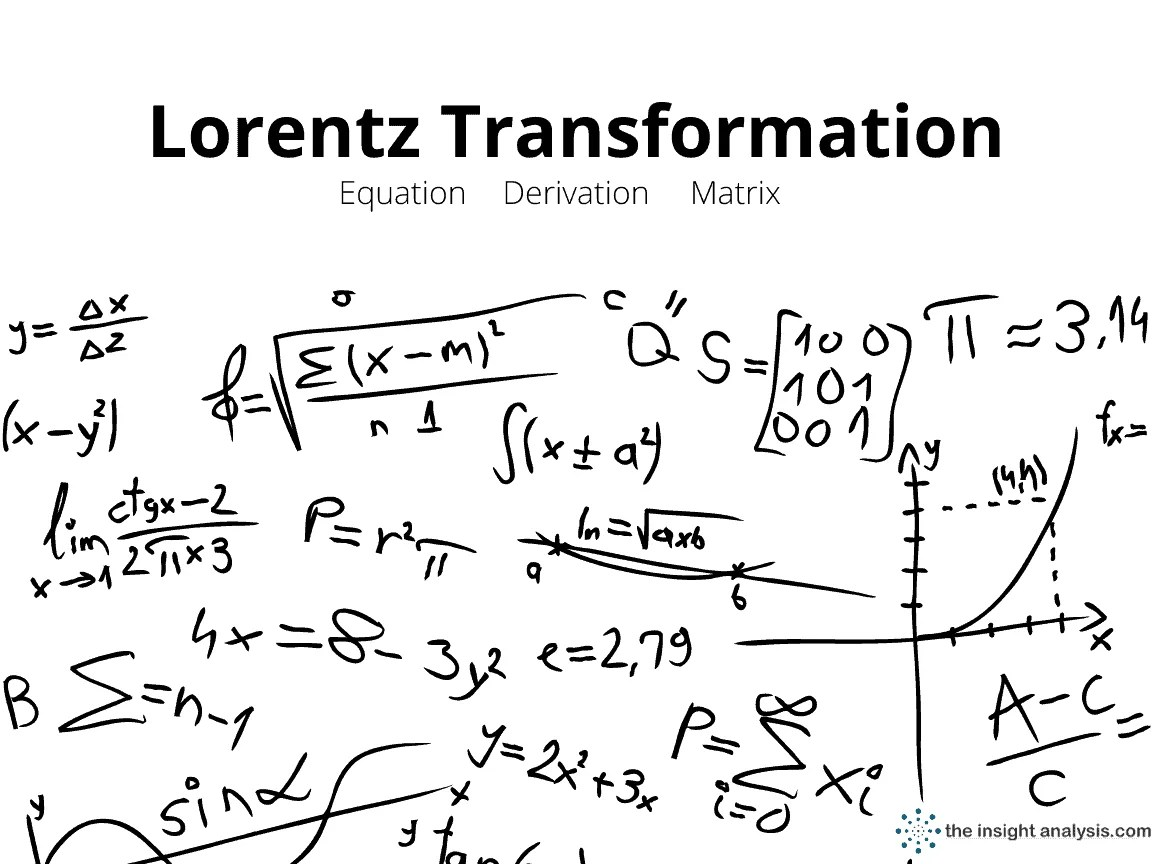Lorentz transformations, within the theory of special relativity, are a set of relationships that account for how the measurements of a physical magnitude obtained by two different observers are related. These relationships established the mathematical basis for Einstein’s theory of special relativity since the Lorentz transformations specify the type of space-time geometry required by Einstein’s theory.

Relativity from Lorentz to EinsteinBuy on Amazon- https://amzn.to/3sGDHSp

Historically the Lorentz transformations were introduced by Hendrik Antoon Lorentz (1853 – 1928), who had introduced them phenomenally to resolve certain inconsistencies between electromagnetism and classical mechanics. Lorentz had discovered in 1900 that Maxwell’s equations were invariant under this set of transformations, now called Lorentz transformations. Like the other physicists, before the development of the theory of relativity, he assumed that the invariant speed for the transmission of electromagnetic waves referred to the transmission through a privileged reference system, a fact known by the name of ether hypotheses.

However, the Lorentz transformations were published in 1904 but his initial mathematical formalism was incorrect. The French mathematician Poincaré developed the set of equations in the consistent way in which they are known today. The works of Minkowski and Poincaré showed that Lorentz relations could be interpreted as the transformation formulas for rotation in fourth-dimensional space-time, which had been introduced by Minkowski.

The shape of Lorentz Transformations relates the measurements of a physical magnitude made by two different inertial observers, being the relativistic equivalent of the Galileo transformation used in physics up to that time.

The Lorentz transformation allows preserving the value of the constant speed of light for all inertial observers.

Coordinate Lorentz Transformations one of the consequences that —unlike what happens in classical mechanics— in relativistic mechanics, there is no absolute time, is that both the time interval between two events and the effective distances measured by different Observers in different states of motion are different. That implies that the time and space coordinates measured by two inertial observers differ from each other. However, due to the objectivity of physical reality, the measurements of both observers are related by fixed rules: the Lorentz transformations for the coordinates.

To examine concretely taking these transformations of coordinates is considered two systems Inertial or observers reference: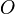and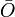and assume that each represents one event S or point of space-time (representable by an instant of time and three spatial coordinates) by two different coordinate systems:

Since the two sets of four coordinates represent the same point in space-time, they must be somehow relatable. The Lorentz transformations say that if the systemis in uniform motion at speedalong the X-axis of the systemand at the initial moment (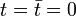) the origin of the coordinates of both systems coincide, then the coordinates attributed by the two observers are related by the following expressions:

Where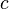is the speed of light in vacuum. The previous relations can also be written in matrix form:

Where the Lorentz factor and the relative speed with respect to light have been introduced to abbreviate the expressions:

The Lorentz transformation above takes this form on the assumption that the coordinate origin of both reference systems is the same for t = 0; if this restriction is removed the concrete form of the equations becomes more complicated. If, in addition, the restriction that the relative speed between the two systems occurs along the X axis and that the axes of both coordinate systems are parallel is eliminated, the expressions of the Lorentz transformation are further complicated, denominating the expression general transformation of Poincaré.

### Lorentz transformations for Momentum and Energy

The covariance requirement of relativity theory requires that any vector magnitude of Newtonian mechanics be represented in relativistic mechanics by a quadrivector or quadristor in relativity theory. Thus, the linear moment requires to be extended to a quadrivector called the energy-moment quadrivector or quadrimotor , which is given by four components, a temporal component (energy) and three spatial components (linear moments in each coordinate direction):

When examining the quads measured by two inertial observers, they are found to measure different components of the moment according to their velocity relative to the observed particle (something that also happens in Newtonian mechanics). If we denote the quadrometer measured by two inertial observersandwith Cartesian coordinate systems of parallel axes and in relative movement along the X axis, such as those considered in the previous section, the quadrometers measured by both observers are related by a transformation of Lorentz given by:

And the inverse transformation is similarly given by:

Or equivalently in matrix form the two previous sets of equations are represented as:

Where the Lorentz factor and the relative speed with respect to light have been entered again for short.

### Lorentz transformations for Quadrivectors

Until now, only inertial systems have been considered in relative motion with respect to the X-axis, but equally, parallel axis systems could have been considered with respect to the Y and Z axes, and in this case, the transformation matrices of coordinates would be given by similar matrices to those considered in the previous sections

The above transformations are sometimes called boosts , space-time rotations, or sometimes Lorentz transformations themselves. The product of any number of transformations of the above type also constitutes a Lorentz transformation. All these products make up a subgroup of the Lorentz group itself. In general, the Lorentz group itself consists of:

• Spatio-temporal rotations or boosts , which can be written as the product of a finite number of boosts of type [*].
• Spatial rotations, consisting of an axis rotation. This type of transformation is also part of the Galileo group.

If improper transformations such as time inversions and spatial reflections are added to these proper transformations , the complete Lorentz group results, consisting of four related components, each homeomorphic to the proper Lorentz group. Once the Lorentz group has been defined, we can write the most general linear transformations possible between measurements taken by inertial observers whose coordinate axes coincide at the initial instant:

Where in addition to the boost given by the coordinate transformation according to the relative separation speed, the two rotations in terms of Euler’s angles have been included:

• The matrix R (θ 1 ,θ 2 ,θ 3 ) aligns the first coordinate system such that the transformed X axis becomes parallel to the separation speed of the two systems.
• The matrix R (φ 1 ,φ 2 ,φ 3 ) is the inverse rotation of which would align the X axis of the second observer with the separation speed.

In more compact form we can write the last transformation in tensor form using the Einstein summation convention as:

General tensor form of Lorentz transformations

Now suppose that instead of measuring vector quantities two observers start to measure the components of some other tensor quantity, suppose that the observersandmeasure in their coordinate systems the same tensor quantity but each one has its own system of coordinates reaching:

The postulate that there is an objective reality independent of the observers and that their measurements can be compared by means of the appropriate covariance transformations leads to the fact that if these observers are inertial, their measurements will be related by the following relationships:

Bibliography & Reference:

Wikipedia.org

encyclopedia.us

Original Article on Relativity published at the Autonomous University of Madrid

Recommended Books

The Mechanics of Lorentz Transformations

Buy on Amazon- https://amzn.to/3dhCz0oGo to Amazon